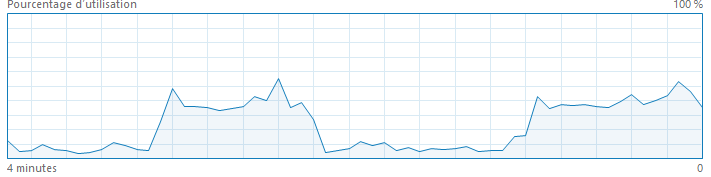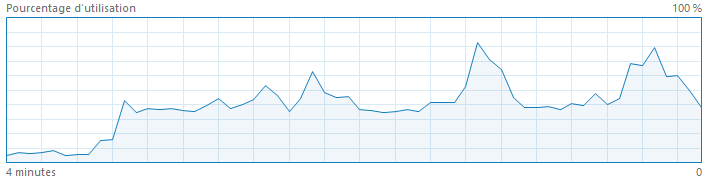# Nearest Neighbours and Sparse Features¶

While trying to apply k-nearest neighbours classifier database, we might face a tricky issue. Let's try to find out what it is, why it is happening and how to solve it.

In :
from jyquickhelper import add_notebook_menu

c:\python370_x64\lib\site-packages\ipykernel\parentpoller.py:116: UserWarning: Parent poll failed.  If the frontend dies,
the kernel may be left running.  Please let us know
ipython-dev@scipy.org
ipython-dev@scipy.org""")

Out:
run previous cell, wait for 2 seconds

## Get the data¶

We use the package mnist.

In :
import mnist
train_images = mnist.train_images()
train_labels = mnist.train_labels()
test_images = mnist.test_images()
test_labels = mnist.test_labels()

In :
train_images.shape, train_labels.shape

Out:
((60000, 28, 28), (60000,))
In :
train_X = train_images.reshape((train_images.shape, train_images.shape * train_images.shape))
test_X = test_images.reshape((test_images.shape, test_images.shape * test_images.shape))

In :
train_X.shape, train_labels.shape

Out:
((60000, 784), (60000,))
In :
train_X[:2]

Out:
array([[0, 0, 0, ..., 0, 0, 0],
[0, 0, 0, ..., 0, 0, 0]], dtype=uint8)

## Train a classifier¶

In :
from sklearn.neighbors import KNeighborsClassifier
knn = KNeighborsClassifier(algorithm="kd_tree")
knn

Out:
KNeighborsClassifier(algorithm='kd_tree', leaf_size=30, metric='minkowski',
metric_params=None, n_jobs=1, n_neighbors=5, p=2,
weights='uniform')
In :
knn.fit(train_X, train_labels)

Out:
KNeighborsClassifier(algorithm='kd_tree', leaf_size=30, metric='minkowski',
metric_params=None, n_jobs=1, n_neighbors=5, p=2,
weights='uniform')

The memory consumption is quite huge. The first hill is training, the second one is the beginning of testing.

In :
from pyquickhelper.helpgen.utils_sphinx_config import NbImage
NbImage("images/train.png")

Out:## Predict¶

In :
# do not do it, it takes for ever.
# yest = knn.predict(test_X)

In :
NbImage("images/test.png")

Out:## Enigma¶

The process almost does not end. We chose a k-d tree to optimize the neighbours search. Why does it take so much memory and so much time? What would you do to optimize it?

In :